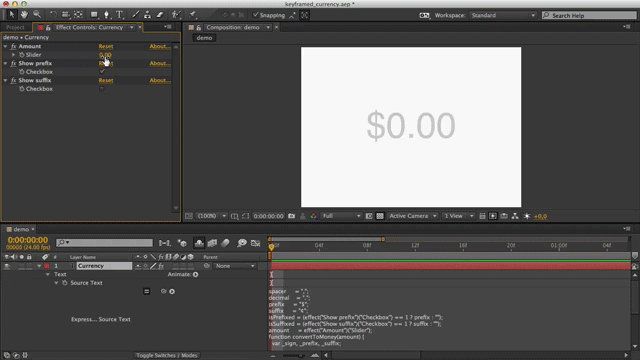## How to format a slider control value as money in After Effects

Written on 25 March 2014, 02:16am under Techniques

Tagged with: ,

Displaying a dynamic amount of money in After Effect can quickly become a bit of a headache. Handling the various mathematic and typographic technicalities of currencies in one bulletproof expression is tricky, but not impossible.

Here’s the result before we go over how it works. Check this Youtube version for a more high definition.The amount of money comes from a linked slider control and is then prefixed or suffixed with a specified currency.

## Supporting currencies and formatting differences

There are various ways to typographically format an amount of money. It differs according to language and geographic location. The formatting differences between french and english for the \$ currency are a good example.

English: `\$10,438,239.43`
French: `10 438 239,43 \$`

Therefore, variables are defined at the beginning of the expression. The default settings display dollars in english.

```spacer     = ",";
decimal    = ".";
prefix     = "\$";
suffix     = "¢";```

But adapting them for a french formatting would be fairly simple.

```spacer     = " ";
decimal    = ",";
prefix     = " \$";
suffix     = "¢";```

## How to use it

1. Create a text layer
1. A Slider Control effect named: Amount
2. A Checkbox Control effect named: Show prefix
3. A Checkbox Control effect named: Show suffix
3. In the text layer’s Source Text property, add this expression:
```spacer     = " ";
decimal    = ".";
prefix     = "\$";
suffix     = "¢";

isPrefixed = (effect("Show prefix")("Checkbox") == 1 ? prefix : "");
isSuffixed = (effect("Show suffix")("Checkbox") == 1 ? suffix : "");
amount     = effect("Amount")("Slider");

function convertToMoney(amount) {
var _sign, _prefix, _suffix;
_sign   = "";
_prefix = ((isPrefixed) ? prefix : "");
_suffix = ((isSuffixed) ? suffix : "");

// Removing the negative
if (amount < 0) {
_sign = "-";
amount = -amount;

}

amount = parseFloat(amount).toFixed(2);

amount = amount.toString().replace(/./g, function(character, index, array) {
if(character === ".") {
return decimal;
} else if ((array.length - index) % 3 || index === 0) {
return character;
} else {
return spacer + character;
}
});

return _sign + _prefix + amount + _suffix;
}

convertToMoney(amount);```

There are definitely many ways to get this kind of result in After Effects, but I thought it would make sense to write mine down and share it here. Do you use a different (maybe simpler) expression to tackle this?